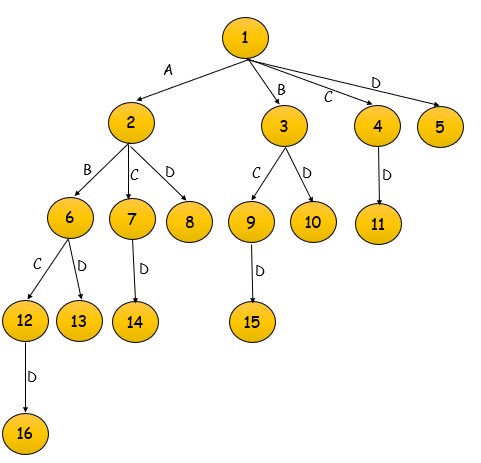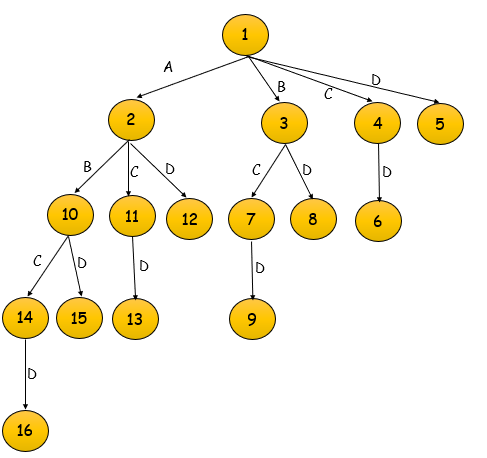×

Search anything:

# Branch and Bound Technique

#### Algorithms branch and boundWith this article, you have explained the idea of Branch and Bound Technique, types of Branch and Bound Technique and applications of the technique.

Contents

1. Introduction to Branch and Bound Technique
2. The Algorithm: Branch and Bound Technique
3. Types of Solution
4. Types of Branch and Bound
5. Why use Branch and Bound?
6. Problems that can be solved using Branch and Bound

## Introduction to Branch and Bound Technique

The Branch and Bound Technique is a problem solving strategy, which is most commonly used in optimization problems, where the goal is to minimize a certain value. The optimized solution is obtained by means of a state space tree (A state space tree is a tree where the solution is constructed by adding elements one by one, starting from the root. Note that the root contains no element). This method is best when used for combinatorial problems with exponential time complexity, since it provides a more efficient solution to such problems.

## The Algorithm: Branch and Bound Technique

In this technique, the first step is to create a function U (which represents an upper bound to the value that node and its children shall achieve), that we intend to minimize. We call this function the objective function. Note that the branch and bound technique can also be used for maximization problems, since multiplying the objective function by -1 coverts the problem to a minimization problem. Let this function have an initial maximum value, according to the conditions of the given problem. Also, let U0 be the initial value of U. We also calculate a cost function C which will give us the exact value that the particular node shall achieve.

The next question is the order in which the tree is to be searched. For this, there exist multiple types of branch and bound, which we shall discuss in detail later. For now, let us assume that there is a set S consisting of subsets of the given set of values in the order in which they need to be searched.

The algorithm of the branch and bound method for this problem will be as follows:

For each subset s in S, do the following:

1. Calculate the value of U(s).
2. If U(s) U0, then U0 = U(s).

In the end, the the subset s for which the current value of U0 is obtained will be the best solution to the given problem, and the value of the cost function at that node will give us the solution. Here, after each level, the value of U0 tells us that there shall be a node with cost less than that value.

## Types of Solutions

For a branch and bound problem, there are two ways in which the solution can be represented:

1. Variable size solution: This solution provides the subset of the given set that gives the optimized solution for the given problem. For example, if the we are to select a combination of elements from {A, B, C, D, E} that optimizes the given problem, and it is found that A, B, and E together give the best solution, then the solution will be {A, B, E}.

2. Fixed size solution: This solution is a sequence of 0s and 1s, with the digit at the ith position denoting whether the ith element should be included or not. Hence, for the earlier example, the solution will be given by {1, 1, 0, 0, 1}.

## Types of Branch and Bound

There are multiple types of the Branch and Bound method, based on the order in which the state space tree is to be searched. We shall now discuss each of these methods in detail. We will be using the variable solution method to denote the solutions in these methods.

## FIFO Branch and Bound

The First-In-First-Out approach to the branch and bound problem follows the queue approach in creating the state-space tree. Here, breadth first search is performed, i.e., the elements at a particular level are all searched, and then the elements of the next level are searched, starting from the first child of the first node in the previous level.

For a given set {A, B, C, D}, the state space tree will be constructed as follows :Here, note that the number assigned to the node signifies the order in which the tree shall be constructed. The element next to the set denotes the next element to be added to the subset. Note that if an element is getting added, it is assumed here that all elements in the set preceeding that element are not added. For example, in node 4, D is getting added. This implies that elements A, B and C are not added.

## LIFO Branch and Bound

The Last-In-First-Out approach to this problem follows the stack approach in creating the state space tree. Here, when nodes get added to the state space tree, think of them as getting added to a stack. When all nodes of a level are added, we pop the topmost element from the stack and then explore it. Hence, the state space tree for the same example {A, B, C, D} will be as follows:Here, one can see that the main difference lies in the order in which the nodes have been explored.

## Least Cost-Branch and Bound

This method of exploration uses the cost function in order to explore the state space tree. Although the previous two methods calculate the cost function at each node, this is not used as a criterion for further exploration.

In this method, after the children of a particular node have been explored, the next node to be explored would be that node out of the unexplored nodes which has the least cost. For example, in the previous example, after reaching node 5, the next node to be explored would be that which has the least cost among nodes 2, 3, 4, 5.

## Why use Branch and Bound?

The Branch and Bound method is preferred over other similar methods such as backtracking when it comes to optimization problems. Here, the cost and the objective function help in finding branches that need not be explored.

Suppose the cost of a particular node has been determined. If this value is greater than that of U0, this means that there is no way this node or its children shall give a solution. Hence, we can kill this node and not explore its further branches. This method helps us rule out cases not worth exploring, and is therefore more efficient.

## Problems that can be solved using Branch and Bound

The Branch and Bound method can be used for solving most combinatorial problems. Some of these problems are given below:

1. Job Sequencing: Suppose there is a set of N jobs and a set of N workers. Each worker takes a specific time to complete each of the N jobs. The job sequencing problem deals with finding that order of the workers, which minimizes the time taken to complete the job.

2. 0/1 Knapsack problem: Given a set of weights of N objects and a sack which can carry a maximum of W units. The problem deals with finding that subset of items such that the maximum possible weight is carried in the sack. Here, one cannot take part of an object, i.e., one can either take an object or not take it. This problem can also be solved using the backtracking, brute force and the dynamic programming approach.

3. Traveling Salesman Problem: Here, we are given a set of N cities, and the cost of traveling between all pairs of cities. The problem is to find a path such that one starts from a given node, visits all cities exactly once, and returns back to the starting city.

With this article at OpenGenus, you must have the complete idea of Branch and Bound Technique.#### Aravind Mohandas

Aravind Mohandas is a Master's student at Indian Institute of Technology, Ropar with B.Sc in Computer Science from Christ University, Bangalore. He is an intern at OpenGenus.

Branch and Bound Technique